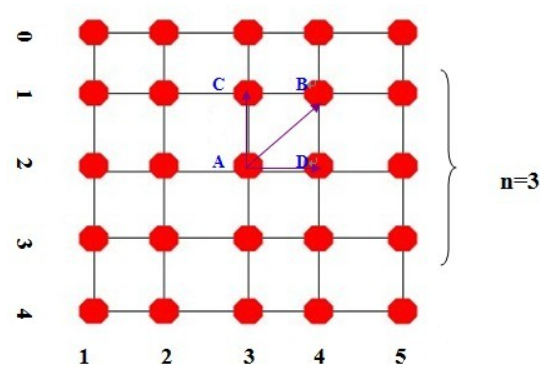# POJ 1408 - Fishnet

## 解题思路

1. 叉积（规范相交）
2. 多边形分解
3. 三角形基于计算几何的面积公式（注意正负）

`n=3` 为例画图 （当然实际上内部的线不一定是正交的，这里只是为了简单说明）## AC 源码

``````//Memory Time
//544K   16MS

#include<iostream>
#include<cmath>
#include<iomanip>
using namespace std;

typedef class Node
{
public:
double x,y;
}location;

double det(double x1,double y1,double x2,double y2)
{
return x1*y2-x2*y1;
}

double cross(location A,location B,location C,location D)       //计算 AB x CD
{
return det(B.x-A.x , B.y-A.y , D.x-C.x , D.y-C.y);
}

/*Compute Intersection*/

double xx,yy;  //坐标返回值
void intersection(location A,location B,location C,location D)
{
double area1=cross(A,B,A,C);
double area2=cross(A,B,A,D);

xx=(area2*C.x - area1*D.x)/(area2-area1);    //本题所求的交点一定是规范相交所得，因此无需判断是否规范相交
yy=(area2*C.y - area1*D.y)/(area2-area1);
return;
}

/*Compute Area*/

double area(location A,location B,location C,location D)
{
double triangle1=fabs(0.5*cross(A,B,A,C));    //用计算几何的方法计算的面积是有向面积
double triangle2=fabs(0.5*cross(A,B,A,D));    //即算出来的面积可能为负数，因此必须用绝对值
// fabs() 为取double的绝对值函数
return triangle1+triangle2;
}

int main(int i,int j,int k)
{
int n;
while(cin>>n)
{
if(!n)
break;

/*Initial*/

location** node=new location*[n+2];
node=new location[n+2];   //下边
node[n+1]=new location[n+2]; //上边

/*Input Down-edge*/

node.x = node.y =0.0;
for(i=1;i<=n;i++)
{
cin>>node[i].x;
node[i].y=0.0;
}
node[n+1].x=1.0;
node[n+1].y=0.0;

/*Input Up-edge*/

node[n+1].x=0.0;
node[n+1].y=1.0;
for(i=1;i<=n;i++)
{
cin>>node[n+1][i].x;
node[n+1][i].y=1.0;
}
node[n+1][n+1].x=1.0;
node[n+1][n+1].y=1.0;

/*Input Left-edge*/

for(i=1;i<=n;i++)
{
node[i]=new location[n+2];
cin>>node[i].y;
node[i].x=0.0;
}

/*Input right-edge*/

for(i=1;i<=n;i++)
{
cin>>node[i][n+1].y;
node[i][n+1].x=1.0;
}

/*Compute Intersection*/

for(j=1;j<=n;j++)
for(i=1;i<=n;i++)
{
intersection(node[j],node[n+1][j],node[i],node[i][n+1]);
node[i][j].x=xx;
node[i][j].y=yy;
}

/*Compute Area*/

double max_area=0.0;

for(i=1;i<=n+1;i++)
for(j=1;j<=n+1;j++)
{
double temp=area(node[i-1][j-1],node[i][j],node[i][j-1],node[i-1][j]);
if(max_area < temp)
max_area = temp;
}

/*Output*/

cout<<fixed<<setprecision(6)<<max_area<<endl;

/*Realx Room*/

delete[] node;
}
return 0;
}``````

目录